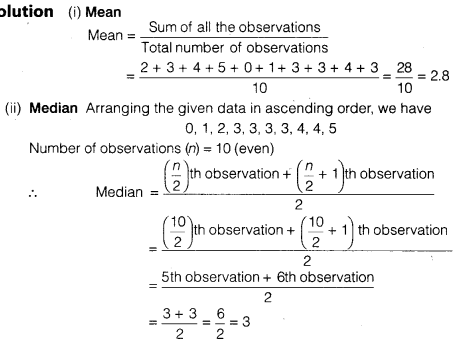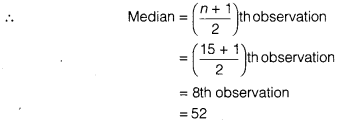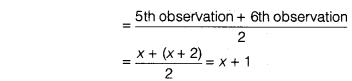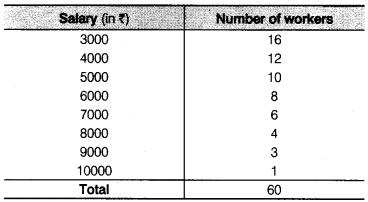## NCERT Solutions for Class 9 Maths Chapter 14 Statistics Ex 14.4

NCERT Solutions for Class 9 Maths Chapter 14 Statistics Ex 14.4 are part of NCERT Solutions for Class 9 Maths. Here we have given NCERT Solutions for Class 9 Maths Chapter 14 Statistics Ex 14.4.

 Board CBSE Textbook NCERT Class Class 9 Subject Maths Chapter Chapter 14 Chapter Name Statistics Exercise Ex 14.4 Number of Questions Solved 6 Category NCERT Solutions

## NCERT Solutions for Class 9 Maths Chapter 14 Statistics Ex 14.4

Question 1.
The following number of goals were scored by a team in a series of 10 matches
2, 3, 4, 5, 0, 1, 3, 3, 4, 3.
Find the mean, median and mode of these scores.
Solution:(iii) Mode Arranging the given data in ascending order, we have 0, 1,2, 3, 3, 3, 3, 4, 4, 5.
Here, 3 occurs most frequently (4 times)
∴ Mode = 3

Question 2.
In a mathematics test given to 15 students, the following marks (out of 100) are recorded
41, 39, 48, 52, 46, 62, 54, 40, 96, 52, 98, 40, 42, 52, 60
Find the mean, median and mode of this data.
Solution:
(i) Mean(ii) Median Arranging the given data in descending order, we have 98, 96, 62, 60, 54, 52, 52, 52, 48, 46, 42, 41,40, 40, 39
Number of observations (n) = 15 which is odd.(iii) Mode Arranging the data in descending order, we have 98, 96, 62, 60, 54, 52, 52, 52, 48, 46, 42, 41,40, 40, 39.
Here, 52 occurs most frequently (3 times).
∴ Mode = 52

Question 3.
The following observations have been arranged in ascending order. If the median of the data is 63, find the value of x.
29, 32, 48, 50, x, x + 2, 72, 78, 84, 95
Solution:
Number of observations (n) = 10which is even.According to question, median = 63
∴ x + 1 = 63 ⇒ x = 63 – 1 = 62
Hence, the value of x is 62.

Question 4.
Find the mode of 14, 25,14, 28,18,17,18,14, 23, 22,14 and 18.
Solution:
The given data is,
14, 25, 14, 28, 18, 17, 18, 14, 23, 22, 14, 18
Arranging the data in ascending order, we have
14, 14, 14, 14, 17, 18, 18, 18, 22, 23, 25, 28
Here, 14 occurs most frequently (4 times).
∴ Mode = 14

Question 5.
Find the mean salary of 60 workers of a factory from the following tableSolution:Hence, the mean salary is ₹ 5083.33.

Question 6.
Give one example of a situation in which
(i) the mean is an appropriate measure of central tendency.
(ii) the mean is not an appropriate measure of central tendency but the median is an appropriate measure of central tendency.
Solution:
(i) Mean marks in a test in mathematics,
(ii) Average beauty

We hope the NCERT Solutions for Class 9 Maths Chapter 14 Statistics Ex 14.4 help you. If you have any query regarding NCERT Solutions for Class 9 Maths Chapter 14 Statistics Ex 14.4, drop a comment below and we will get back to you at the earliest.